# M-Ploding Polytopes

Here are some thoughts on making some cool polytope objects. It's long but hopefully accessible ( but maybe a bit boring) and has some nice pictures.

# Intro

Have you heard of the dual of a regular polyhedra?

The dual of a regular polyhedra, $R$, can be made by taking the centre of each face of $R$ and turning it into a vertex. Then we join these new vertices if and only if the faces they are the centre of share an edge.

For example, take a cube. Imagine a vertex in the centre of each square face. Now join up these vertices if and only if the square faces they are on touch. What do you get?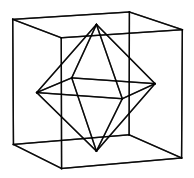We get this diamond looking shape called an octahedron!

Now, what if we begun with this octahedron to begin with and took the dual of it?

We'd get a cube again! In fact no matter what Regular Polyhedron we start with we always find that the Dual of the Dual of it is itself in terms of how it is connected. (*Why is this True? *) So the operation of dualling a regular polyhedron repeats itself after every two operations when thinking of the type of regular polyhedron it produces.

# Another way of Dualling

Now let's think of the idea of dualling something in a different way. If we take the $3D$ co-ordinates of a face of regular polyhedron $(v_1, v_2, ..., v_s)$ and take take the mean value of them $\frac{v_1 + v_2 + \cdots + v_s }{s}$ then this gives the centre of that shape. (*Why is this True? *)

Now if we take convex hull of all the mean values of each face's vertices we find that we get the dual of the regular polyhedron too!

So what did we do?

1. We take the $\color{#3D99F6}{\text{the vertices}}$ on the faces of the original regular polyhedron.

2. We take their mean to make new vertices.

3. Then we take the convex hull of these new vertices.

Now what if we changed step 1 to

We take the the vertices of $\color{#3D99F6}{\text{the edges}}$ of the original polyhedron?

# A First Generalisation

Let's start off with a cube and apply this new algorithm using the mean of the edges over and over again for each regular polyhedron.

Cube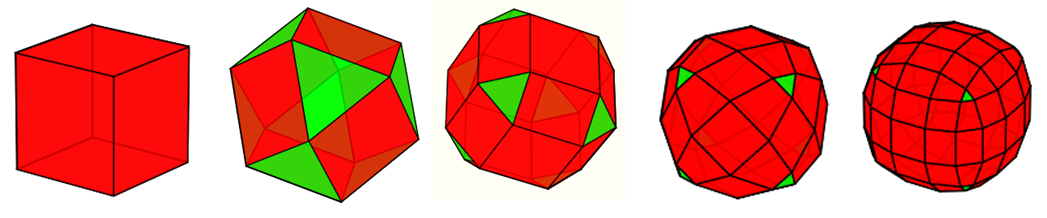Octahedron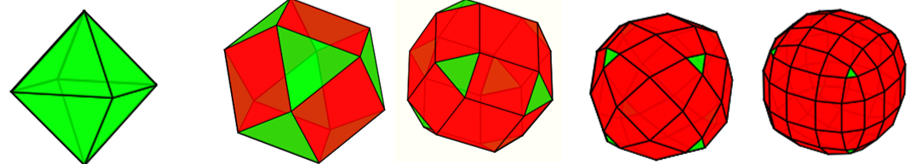Tetrahedron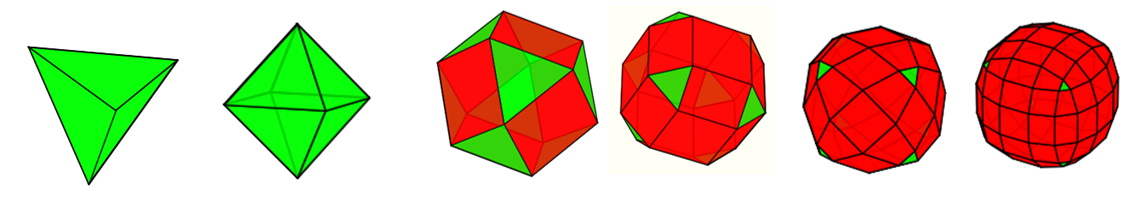Dodecahedron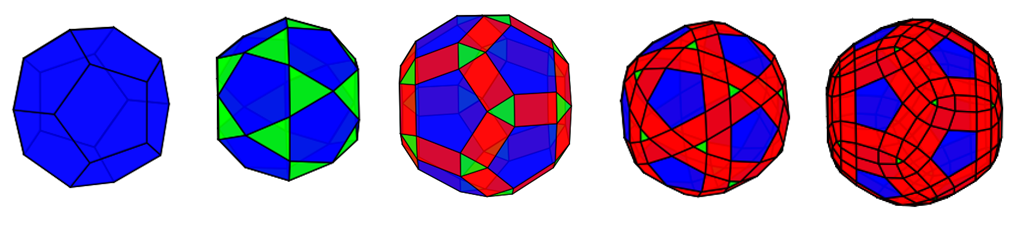Icosahedron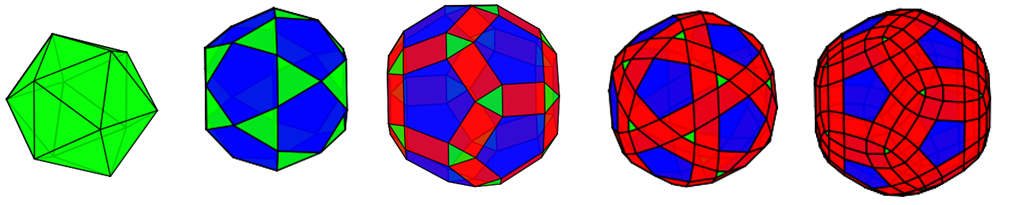# Some questions

It looks as if they all converge respectively to some weird surface.

• What are these surfaces? I don't know exactly (yet)?

• And do they have any interesting properties?

• How do they roll on the ground?

• What way up would the float in water?

• What can we say about their symmetries?

• What formulas describe their surfaces and volumes?

• What are the lines of shortest distances on them?

• Can we assign numbers $1, 2, \ldots, k$ on similar faces with repetition to make it a fair dice on any of the iterations?

• Are there any applications into computer graphics for this kind of method for making smooth approximations of objects iteratively?

• What's a fast algorithm for computing these?

# Another generalisation.

Why did we look at Regular Polyhedra in the first place? What if we take a higher dimensional object, like a 4D hypercube? Then we can take the mean of its other properties (sometimes called manifolds) like its volumes.

• This should generalise nicely. In the last case the regular polyhedra looked as if they tended towards 3 unique surfaces as they approach their limits.

• Will this be true for all regular polytopes (their $n$-dimensional analogues)?

• Given any initial polytope and any manifold we are meaning over, can we deduce the number of vertices, edges , volumes etc given the $k^{th}$ iteration?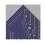Note by Roberto Nicolaides
4 years, 11 months ago

This discussion board is a place to discuss our Daily Challenges and the math and science related to those challenges. Explanations are more than just a solution — they should explain the steps and thinking strategies that you used to obtain the solution. Comments should further the discussion of math and science.

When posting on Brilliant:

• Use the emojis to react to an explanation, whether you're congratulating a job well done , or just really confused .
• Ask specific questions about the challenge or the steps in somebody's explanation. Well-posed questions can add a lot to the discussion, but posting "I don't understand!" doesn't help anyone.
• Try to contribute something new to the discussion, whether it is an extension, generalization or other idea related to the challenge.

MarkdownAppears as
*italics* or _italics_ italics
**bold** or __bold__ bold
- bulleted- list
• bulleted
• list
1. numbered2. list
1. numbered
2. list
Note: you must add a full line of space before and after lists for them to show up correctly
paragraph 1paragraph 2

paragraph 1

paragraph 2

[example link](https://brilliant.org)example link
> This is a quote
This is a quote
    # I indented these lines
# 4 spaces, and now they show
# up as a code block.

print "hello world"
# I indented these lines
# 4 spaces, and now they show
# up as a code block.

print "hello world"
MathAppears as
Remember to wrap math in $$ ... $$ or $ ... $ to ensure proper formatting.
2 \times 3 $2 \times 3$
2^{34} $2^{34}$
a_{i-1} $a_{i-1}$
\frac{2}{3} $\frac{2}{3}$
\sqrt{2} $\sqrt{2}$
\sum_{i=1}^3 $\sum_{i=1}^3$
\sin \theta $\sin \theta$
\boxed{123} $\boxed{123}$

Sort by:

Julian, thanks so much! This is exactly the what I've been looking into but never knew the term :)

- 4 years, 11 months ago

This might help

- 4 years, 11 months ago